Updating search results...

# 119 Results

View
Selected filters:
• Equations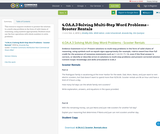Conditional Remix & Share Permitted
CC BY-NC-SA
Rating
0.0 stars

This resource requires students to present the solution to a multi-step problem in the form of valid chains of reasoning, using symbols appropriately. Students must use the four operations with whole numbers to solve problems.

Subject:
Education
Mathematics
Material Type:
Activity/Lab
Assessment
Homework/Assignment
Lesson
Author:
Linda Schoenbrodt
Amanda Ray
KIm Quintyne
10/04/2018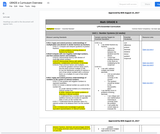Conditional Remix & Share Permitted
CC BY-NC-SA
Rating
0.0 stars

6th Grade Regular Math: Course Scope & Sequence.

Subject:
Mathematics
Material Type:
Full Course
Provider:
Liberty Public Schools
08/15/2017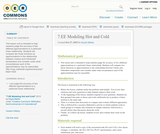Conditional Remix & Share Permitted
CC BY-NC
Rating
4.0 stars

This lesson unit is intended to help students judge the accuracy of two different approximations to a particular linear relationship. Students will compare two linear functions as approximations to the relationship between Celsius and Fahrenheit temperature and consider under what circumstances each of the approximations may be reasonable.

Subject:
Mathematics
Material Type:
Lesson
Author:
Kayla Martin
01/19/2017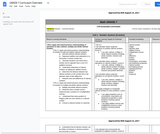Conditional Remix & Share Permitted
CC BY-NC-SA
Rating
0.0 stars

7th Grade Regular Math: Course Scope & Sequence.

Subject:
Mathematics
Material Type:
Full Course
Provider:
Liberty Public Schools
08/15/2017Unrestricted Use
CC BY
Rating
0.0 stars

This task examines the ways in which the plane can be covered by regular polygons in a very strict arrangement called a regular tessellation. These tessellations are studied here using algebra, which enters the picture via the formula for the measure of the interior angles of a regular polygon (which should therefore be introduced or reviewed before beginning the task). The goal of the task is to use algebra in order to understand which tessellations of the plane with regular polygons are possible.

Subject:
Mathematics
Algebra
Geometry
Material Type:
Activity/Lab
Provider:
Illustrative Mathematics
Provider Set:
Illustrative Mathematics
Author:
Illustrative Mathematics
01/21/2013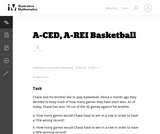Unrestricted Use
CC BY
Rating
0.0 stars

This task provides a simple but interesting and realistic context in which students are led to set up a rational equation (and a rational inequality) in one variable, and then solve that equation/inequality for an unknown variable.

Subject:
Mathematics
Algebra
Material Type:
Activity/Lab
Provider:
Illustrative Mathematics
Provider Set:
Illustrative Mathematics
Author:
Illustrative Mathematics
12/15/2012Conditional Remix & Share Permitted
CC BY-NC-SA
Rating
0.0 stars

This course is designed to introduce students who wish to specialize in stress analysis of thin-walled structures to more advanced topics such as the analysis of statically indeterminate structures, warping, constraint stresses, shear diffusion, and elements of plate bending.

Subject:
Engineering
Material Type:
Full Course
Provider:
Delft University of Technology
Provider Set:
Delft University OpenCourseWare
Author:
Dr. Mostafa Abdalla
02/07/2014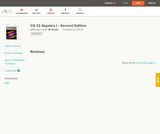Conditional Remix & Share Permitted
CC BY-NC-SA
Rating
2.0 stars

A work in progress, CK-12's Algebra I Second Edition is a clear presentation of algebra for the high school student. Topics include: Equations and Functions, Real Numbers, Equations of Lines, Solving Systems of Equations and Quadratic Equations.

Subject:
Algebra
Functions
Material Type:
Textbook
Provider:
CK-12 Foundation
Provider Set:
CK-12 FlexBook
Author:
Andrew
Annamaria
Anne
Eve
Farbizio
Gloag
Rawley
12/03/2010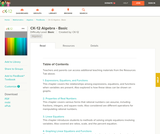Conditional Remix & Share Permitted
CC BY-NC-SA
Rating
0.0 stars

CK-12 Foundation's Basic Algebra FlexBook is an introduction to the algebraic topics of functions, equations, and graphs for middle-school and high-school students.

Subject:
Algebra
Functions
Material Type:
Textbook
Provider:
CK-12 Foundation
Provider Set:
CK-12 FlexBook
Author:
Farbizio, Annamaria
Gloag, Andrew
Gloag, Anne
Kramer, Melissa
09/21/2010Conditional Remix & Share Permitted
CC BY-NC-SA
Rating
0.0 stars

This site teaches High Schoolers how to create equations through a series of 298 questions and interactive activities aligned to 5 Common Core mathematics skills.

Subject:
Mathematics
Algebra
Material Type:
Activity/Lab
Interactive
Provider:
Provider Set:
01/09/2015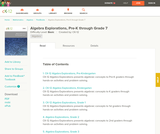Conditional Remix & Share Permitted
CC BY-NC-SA
Rating
0.0 stars

CK-12 Algebra Explorations is a hands-on series of activities that guides students from Pre-K to Grade 7 through algebraic concepts.

Subject:
Algebra
Material Type:
Activity/Lab
Teaching/Learning Strategy
Provider:
CK-12 Foundation
Provider Set:
CK-12 FlexBook
Author:
Carol ­ Findell, Mary ­Cavanagh, Carole ­Greenes
02/23/2012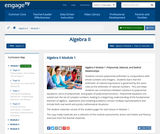Conditional Remix & Share Permitted
CC BY-NC-SA
Rating
0.0 stars

Students connect polynomial arithmetic to computations with whole numbers and integers.  Students learn that the arithmetic of rational expressions is governed by the same rules as the arithmetic of rational numbers.  This unit helps students see connections between solutions to polynomial equations, zeros of polynomials, and graphs of polynomial functions.  Polynomial equations are solved over the set of complex numbers, leading to a beginning understanding of the fundamental theorem of algebra.  Application and modeling problems connect multiple representations and include both real world and purely mathematical situations.

Subject:
Algebra
Material Type:
Module
Provider:
New York State Education Department
Provider Set:
EngageNY
05/14/2013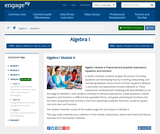Conditional Remix & Share Permitted
CC BY-NC-SA
Rating
0.0 stars

In earlier modules, students analyze the process of solving equations and developing fluency in writing, interpreting, and translating between various forms of linear equations (Module 1) and linear and exponential functions (Module 3). These experiences combined with modeling with data (Module 2), set the stage for Module 4. Here students continue to interpret expressions, create equations, rewrite equations and functions in different but equivalent forms, and graph and interpret functions, but this time using polynomial functions, and more specifically quadratic functions, as well as square root and cube root functions.

Subject:
Algebra
Material Type:
Module
Provider:
New York State Education Department
Provider Set:
EngageNY
09/17/2013Conditional Remix & Share Permitted
CC BY-NC-SA
Rating
0.0 stars

This site teaches Reasoning with Equations and Inequalities to High Schoolers through a series of 5909 questions and interactive activities aligned to 36 Common Core mathematics skills.

Subject:
Mathematics
Algebra
Material Type:
Activity/Lab
Interactive
Provider:
Provider Set:
01/09/2015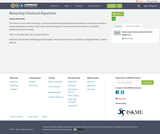Conditional Remix & Share Permitted
CC BY-NC
Rating
0.0 stars

This lesson is one to two hours long. It assumes students have already learned the basics of molecules and atoms. Using manipulatives, students learn what a balanced equation looks like and how to balance an equation. Additional links are included.There is a teacher plan and a student handout.Materials: colored marshmallows/gumdrops/paper circles (at least 4 colors), toothpicks or spaghetti sticks, napkins optional.

Subject:
Applied Science
Material Type:
Lesson Plan
Author:
Shana Friend
03/26/2019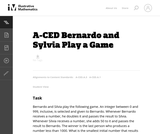Unrestricted Use
CC BY
Rating
0.0 stars

This task presents a simple but mathematically interesting game whose solution is a challenging exercise in creating and reasoning with algebraic inequalities. The core of the task involves converting a verbal statement into a mathematical inequality in a context in which the inequality is not obviously presented, and then repeatedly using the inequality to deduce information about the structure of the game.

Subject:
Mathematics
Algebra
Material Type:
Activity/Lab
Provider:
Illustrative Mathematics
Provider Set:
Illustrative Mathematics
Author:
Illustrative Mathematics
10/21/2013Unrestricted Use
CC BY
Rating
0.0 stars

The emphasis in this task is on the progression of equations, from two that involve different values of the sales tax, to one that involves the sales tax as a parameter. It is designed to foster the habit of looking for regularity in solution procedures, so that students don't approach every equation as a new problem but learn to notice familiar types.

Subject:
Mathematics
Algebra
Material Type:
Activity/Lab
Provider:
Illustrative Mathematics
Provider Set:
Illustrative Mathematics
Author:
Illustrative Mathematics
05/01/2012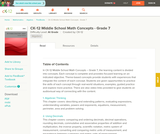Conditional Remix & Share Permitted
CC BY-NC-SA
Rating
0.0 stars

CK-12 Middle School Math Concepts for seventh grade provides a complete textbook. It presents topics including algebraic thinking, patterns, decimals, decimal operations, fractions, fraction operations, integers, integer operations, ratios, rates, proportions, percents, percent applications, equations, solving equations, inequalities, functions, graphing functions, geometry, plane geometry, solid geometry, area, perimeter, surface area, volume, statistics including mean, median, mode and range, graphing and types of graphs, and probability.

Subject:
Mathematics
Algebra
Geometry
Numbers and Operations
Statistics and Probability
Material Type:
Textbook
Provider:
CK-12 Foundation
Provider Set:
CK-12 FlexBook
Author:
Jen Kershaw
11/30/2012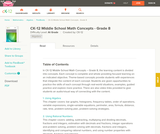Conditional Remix & Share Permitted
CC BY-NC
Rating
0.0 stars

In CK-12 Middle School Math Concepts – Grade 8, the learning content is divided into concepts. Each concept is complete and whole providing focused learning on an indicated objective. Theme-based concepts provide students with experiences that integrate the content of each concept. Students are given opportunities to practice the skills of each concept through real-world situations, examples, guided practice and explore more practice. There are also video links provided to give students an audio/visual way of connecting with the content.

Subject:
Mathematics
Material Type:
Full Course
Lesson Plan
Textbook
Unit of Study
Provider:
CK-12 Foundation
Provider Set:
CK-12 FlexBook
03/28/2016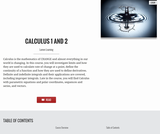Rating
0.0 stars

Calculus is the mathematics of CHANGE and almost everything in our world is changing. In this course, you will investigate limits and how they are used to calculate rate of change at a point, define the continuity of a function and how they are used to define derivatives. Definite and indefinite integrals and their applications are covered, including improper integrals. Late in the course, you will find Calculus with parametric equations and polar coordinates, sequences and series, and vectors.

Subject:
Calculus
Material Type:
Full Course
Textbook
Provider:
Lumen Learning
Provider Set:
Candela Courseware
Author:
Dale Hoffman
David Lippman
Jeff Eldridge
Melonie Rasmussen
03/31/2016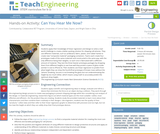Educational Use
Rating
0.0 stars

Students apply their knowledge of linear regression and design to solve a real-world challenge to create a better packing solution for shipping cell phones. They use different materials, such as cardboard, fabric, plastic, and rubber bands to create new “composite material” packaging containers. Teams each create four prototypes made of the same materials and constructed in the same way, with the only difference being their weights, so each one is fabricated with a different amount of material. They test the three heavier prototype packages by dropping them from different heights to see how well they protect a piece of glass inside (similar in size to iPhone 6). Then students use linear regression to predict from what height they can drop the fourth/final prototype of known mass without the “phone” breaking. Success is not breaking the glass but not underestimating the height by too much either, which means using math to accurately predict the optimum drop height.

Subject:
Algebra
Physics
Material Type:
Activity/Lab
Provider:
TeachEngineering
Provider Set:
Activities
Author:
Abbie Morneault
Brett Doudican
Kellee Callahan
08/02/2017Conditional Remix & Share Permitted
CC BY-NC-SA
Rating
0.0 stars

College Algebra is an introductory text for a college algebra survey course. The material is presented at a level intended to prepare students for Calculus while also giving them relevant mathematical skills that can be used in other classes. The authors describe their approach as "Functions First," believing introducing functions first will help students understand new concepts more completely. Each section includes homework exercises, and the answers to most computational questions are included in the text (discussion questions are open-ended).

Subject:
Algebra
Material Type:
Textbook
Provider:
Stitz-Zeager Open Source Mathematics
Author:
Carl Stitz
Jeff Zeager
07/15/2011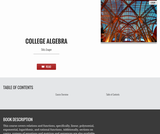Rating
0.0 stars

This course covers relations and functions, specifically, linear, polynomial, exponential, logarithmic, and rational functions. Additionally, sections on conics, systems of equations and matrices and sequences are also available.

Subject:
Algebra
Material Type:
Full Course
Textbook
Provider:
Lumen Learning
Provider Set:
Candela Courseware
Author:
Carl Stitz
Jeff Zeager
03/31/2016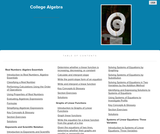Rating
0.0 stars

It is often said that mathematics is the language of science. If this is true, then the language of mathematics is numbers. The earliest use of numbers occurred 100 centuries ago in the Middle East to count, or enumerate items. Farmers, cattlemen, and tradesmen used tokens, stones, or markers to signify a single quantitya sheaf of grain, a head of livestock, or a fixed length of cloth, for example. Doing so made commerce possible, leading to improved communications and the spread of civilization.

Subject:
Algebra
Material Type:
Full Course
Textbook
Provider:
Lumen Learning
Provider Set:
Candela Courseware
08/18/2016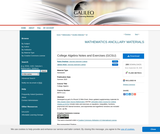Unrestricted Use
CC BY
Rating
0.0 stars

Developed as part of a Round 13 Mini-Grant, these updated supplementary materials for Stitz-Zeager Open Source Mathematics and the LibGuides Open Course for College Algebra at GCSU include notes and exercises on equations, inequalities, functions, polynomial and rational functions, and exponential and logarithmic functions are included in one .zip file.

Subject:
Algebra
Material Type:
Activity/Lab
Lecture Notes
Provider:
Georgia Gwinnett College
Author:
Janice Alves
10/03/2022Only Sharing Permitted
CC BY-NC-ND
Rating
0.0 stars

This lesson unit is intended to help teachers assess how well students are able to use linear inequalities to create a set of solutions. In particular, the lesson will help teachers identify and assist students who have difficulties in: representing a constraint by shading the correct side of the inequality line; and understanding how combining inequalities affects a solution space.

Subject:
Mathematics
Material Type:
Assessment
Lesson Plan
Provider:
Shell Center for Mathematical Education
Provider Set:
Mathematics Assessment Project (MAP)
04/26/2013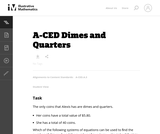Unrestricted Use
CC BY
Rating
0.0 stars

This task does not actually require that the student solve the system but that they recognize the pairs of linear equations in two variables that would be used to solve the system. This is an important step in the process of solving systems.

Subject:
Mathematics
Algebra
Material Type:
Activity/Lab
Provider:
Illustrative Mathematics
Provider Set:
Illustrative Mathematics
Author:
Illustrative Mathematics
05/01/2012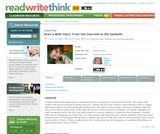Some Rights Reserved
Rating
0.0 stars

Students create math stories by first drawing, then writing, and finally using math symbols to show addition or subtraction.

Subject:
Arts and Humanities
Language, Grammar and Vocabulary
Material Type:
Activity/Lab
Lesson Plan
Provider:
Provider Set:
09/30/2013Only Sharing Permitted
CC BY-NC-ND
Rating
4.5 stars

This lesson unit is intended to help assess how well students are able to interpret and use scale drawings to plan a garden layout. This involves using proportional reasoning and metric units.

Subject:
Algebra
Geometry
Ratios and Proportions
Material Type:
Assessment
Lesson Plan
Provider:
Shell Center for Mathematical Education
Provider Set:
Mathematics Assessment Project (MAP)
04/26/2013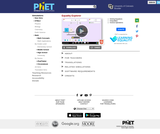Unrestricted Use
CC BY
Rating
0.0 stars

Explore what it means for a mathematical statement to be balanced or unbalanced by interacting with objects on a balance. Discover the rules for keeping it balanced. Collect stars by playing the game!

Subject:
Mathematics
Material Type:
Simulation
Provider:
Provider Set:
PhET Interactive Simulations
Author:
David Wood
Marleene Buttice
Meenakshi Raghavan
03/06/2019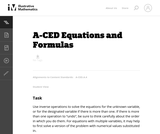Unrestricted Use
CC BY
Rating
0.0 stars

This task asks students to use inverse operations to solve the equations for the unknown variable, or for the designated variable if there is more than one. Two of the equations are of physical significance and are examples of Ohm's Law and Newton's Law of Universal Gravitation.

Subject:
Mathematics
Algebra
Material Type:
Activity/Lab
Provider:
Illustrative Mathematics
Provider Set:
Illustrative Mathematics
Author:
Illustrative Mathematics
05/01/2012
Remix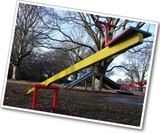Conditional Remix & Share Permitted
CC BY-NC
Rating
0.0 stars

Gallery OverviewAllow students who have a clear understanding of the content thus far in the unit to work on Gallery problems of their choosing. You can then use this time to provide additional help to students who need review of the unit's concepts or to assist students who may have fallen behind on work.Gallery DescriptionsKeep It BalancedStudents will use reasoning to complete some equations to make them true.Equation SortStudents will sort equations into three groups: equations with one solution, equations with many solutions, and equations with no solutionsOn the Number LineStudents will use a number line to identify numbers that make an equation or inequality true.How Many Colors?Students will write and solve an equation to find the number of different colored blocks in a box.Value of sStudents will use a property of equality to solve an equation with large numbers.Marbles in a CupStudents are given information about the weight of a cup with two different amounts of marbles in it. They use this information to find the weight of the cup.When Is It True?Students will use what they know about 0 and 1 to decide when a certain equation is true.

Subject:
Algebra
Material Type:
Lesson Plan
Author:
Amber Delliger
12/12/2017Only Sharing Permitted
CC BY-NC-ND
Rating
3.0 stars

This lesson unit is intended to help teachers assess how well students are able to: use the Pythagorean theorem to derive the equation of a circle; and translate between the geometric features of circles and their equations.

Subject:
Geometry
Material Type:
Assessment
Lesson Plan
Provider:
Shell Center for Mathematical Education
Provider Set:
Mathematics Assessment Project (MAP)
04/26/2013Only Sharing Permitted
CC BY-NC-ND
Rating
0.0 stars

This lesson unit is intended to help teachers assess how well students are able to: translate between the equations of circles and their geometric features; and sketch a circle from its equation.

Subject:
Geometry
Material Type:
Assessment
Lesson Plan
Provider:
Shell Center for Mathematical Education
Provider Set:
Mathematics Assessment Project (MAP)
04/26/2013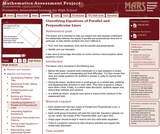Only Sharing Permitted
CC BY-NC-ND
Rating
0.0 stars

This lesson unit is intended to help teachers assess how well students are able to understand the relationship between the slopes of parallel and perpendicular lines and, in particular, to help identify students who find it difficult to: find, from their equations, lines that are parallel and perpendicular; and identify and use intercepts. It also aims to encourage discussion on some common misconceptions about equations of lines.

Subject:
Mathematics
Material Type:
Assessment
Lesson Plan
Provider:
Shell Center for Mathematical Education
Provider Set:
Mathematics Assessment Project (MAP)
04/26/2013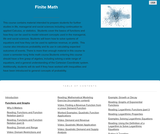Rating
0.0 stars

Mathematics

Subject:
Mathematics
Material Type:
Full Course
Textbook
Provider:
Lumen Learning
Provider Set:
Candela Courseware
03/31/2016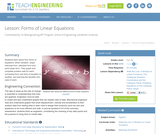Educational Use
Rating
0.0 stars

Students learn about four forms of equations: direct variation, slope-intercept form, standard form and point-slope form. They graph and complete problem sets for each, converting from one form of equation to another, and learning the benefits and uses of each.

Subject:
Engineering
Mathematics
Algebra
Material Type:
Lesson Plan
Provider:
TeachEngineering
Provider Set:
TeachEngineering
Author:
Aubrey McKelvey
09/18/2014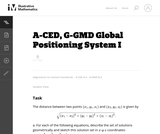Unrestricted Use
CC BY
Rating
0.0 stars

This question examines the algebraic equations for three different spheres. The intersections of each pair of spheres are then studied, both using the equations and thinking about the geometry of the spheres. For two spheres where one is not contained inside of the other there are three possibilities for how they intersect.

Subject:
Mathematics
Algebra
Geometry
Material Type:
Activity/Lab
Provider:
Illustrative Mathematics
Provider Set:
Illustrative Mathematics
Author:
Illustrative Mathematics
01/21/2013Conditional Remix & Share Permitted
CC BY-NC-SA
Rating
0.0 stars

This site teaches High Schoolers how to express geometric properties with equations through a series of 1721 questions and interactive activities aligned to 12 Common Core mathematics skills.

Subject:
Mathematics
Geometry
Material Type:
Activity/Lab
Interactive
Provider:
Provider Set:
01/09/2015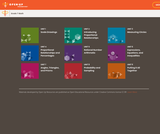Unrestricted Use
CC BY
Rating
0.0 stars

Student-facing 7th grade math resources. Covers scale drawings, proportion, percentages, probability, expressions, and geometry.

Subject:
Mathematics
Material Type:
Unit of Study
Provider:
Open Up Resources